# The spherical space $S^3$

## What is the 3-sphere?

The space $S^3$ is the 3-dimensional analogue of the usual sphere in 2 dimensions. It is an isotropic space (all the directions play the same role). Among the eight Thurston geometries, it is the only compact one. It can be seen as the unit tangent bundle of the 2-sphere. This point of view is also known as the Hopf fibration.

Click on the button below to reveal a concrete model of the 3-sphere.

The 3-sphere is the unit sphere of the 4-dimensional space $\mathbb R^4$. Thus a possible model $X$ is $$X = \left\{ (x,y,z,w) \in \mathbb R^4 \mid x^2 + y^2 + z^2 + w^2 = 1 \right\}.$$ The euclidean metric on $\mathbb R^4$ induces a riemanian metric on $X$ $$ds^2 = dx^2 + dy^2 + dz^2 + dw^2.$$ Its isometry group is $O(4)$, i.e. the group of linear isometries of $\mathbb R^4$ preserving the euclidean metric in $\mathbb R^4$. This group acts transitively on the unit tangent bundle of $X$. Thus $X$ is not only homogeneous but also isotropic.

## Some views of 3-sphere

Warning: Some of the real-time simulations below requires a powerful graphic card. If your computer is not fast enough, you can reduce the size of your browser window. Click on the button below to reveal the fly commands.

The default controls to fly in the scene are the following. You can choose your keyboard in the Option controls pannel in the top right corner of the window

Command QWERTY keyboard AZERTY keyboard
Yaw left a q
Yaw right d d
Pitch up w z
Pitch down s s
Roll left q a
Roll right e e
Move forward arrow up arrow up
Move backward arrow down arrow down
Move to the left arrow left arrow left
Move the the right arrow right arrow right
Move upwards ' ù
Move downwards / =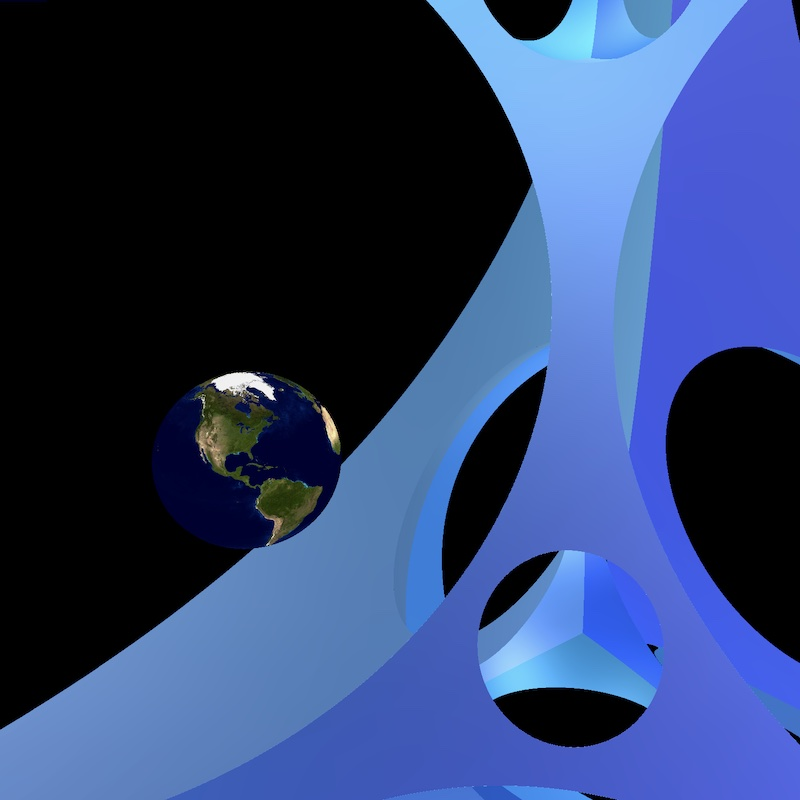##### Virtual reality

VR view of the 3-sphere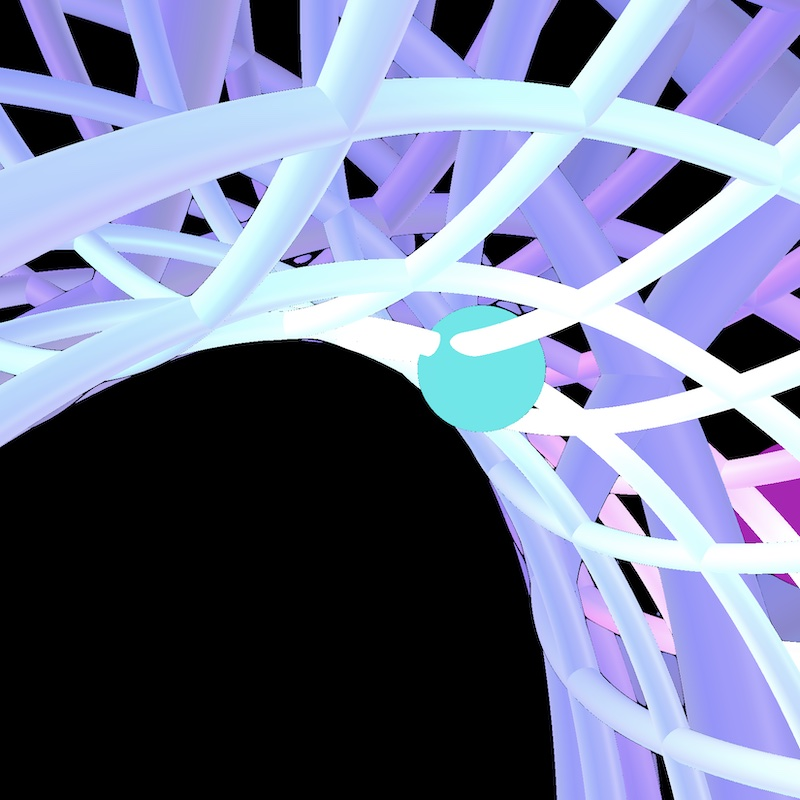##### Clifford torus

Clifford torus made of "vertical" and "horizontal" lines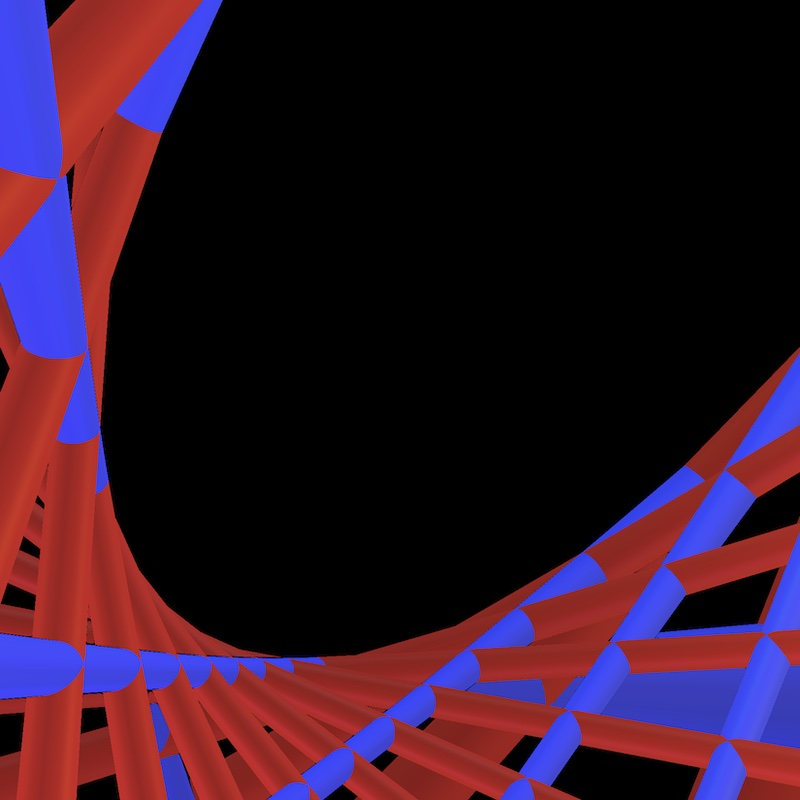##### Clifford torus

Clifford torus made of geodesics corresponding to Villarceau circles

HD pictures of the 3-sphere can be found in the gallery

## Features of 3-sphere

### Geodesic Convergence

Positive curvature makes geodesics which are initially diverging come back together again, and meet at the antipode to their point of origin.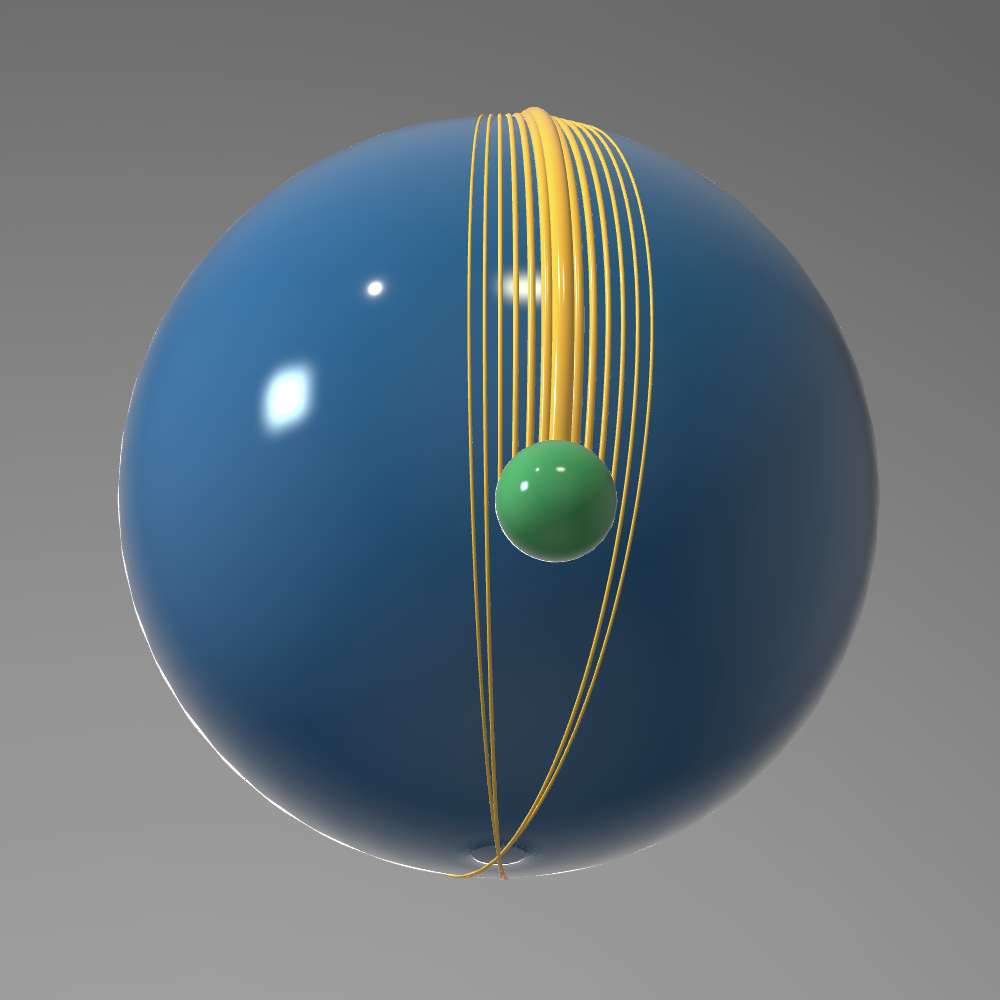##### Geodesics on $S^2$

The Effect of Positive Curvature

### The Hopf Fibration

The Hopf fibration is a decomposition of the 3-sphere as a circle bundle over the two-sphere. The simulation below represents some fibers of the Hopf fibration. They corresponds to the vertices of a regular dodecahedron in $S^2$. A reflecting sphere has been adding to the scene to capture a more global view of these fibers.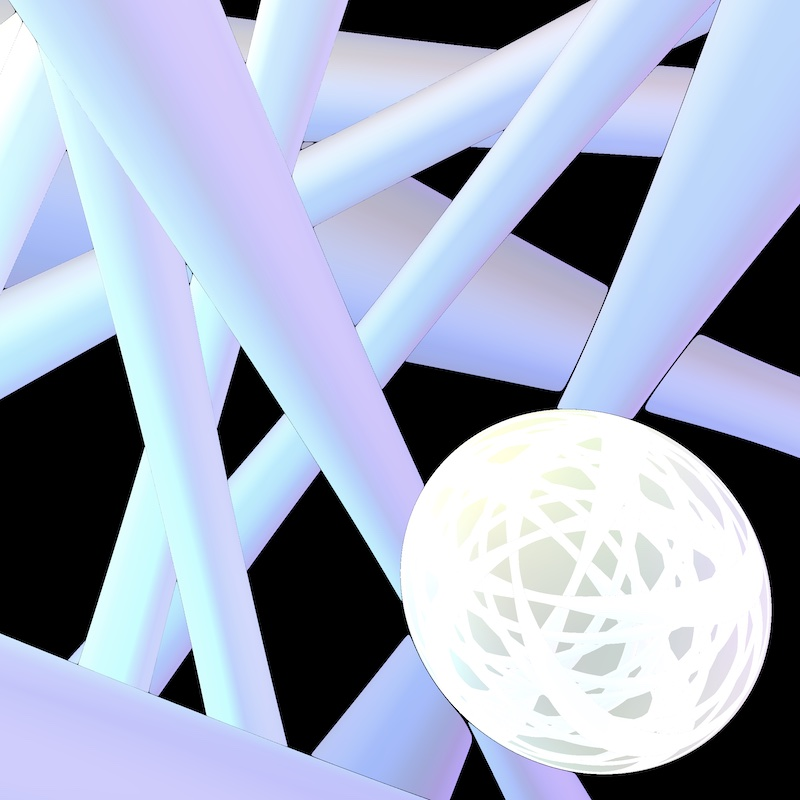##### The Hopf Fibration

An intrinsic view.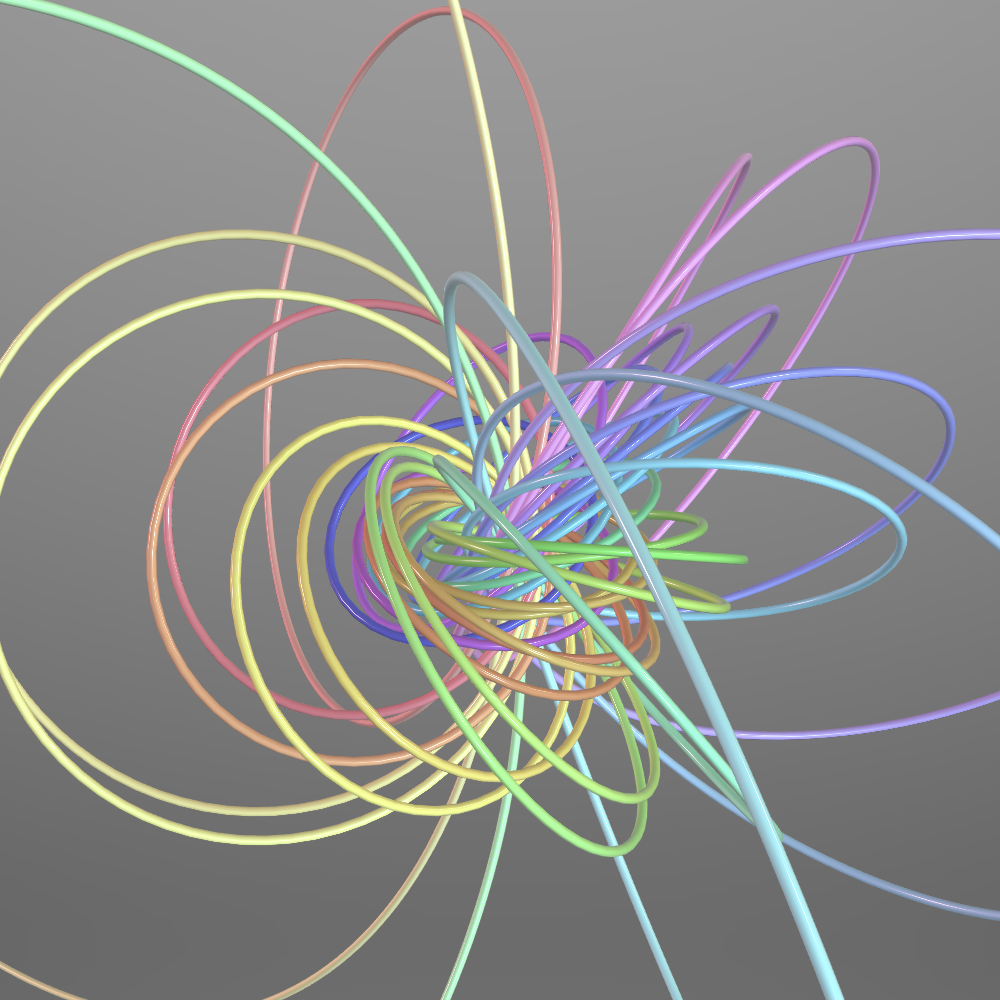##### The Hopf Fibration

An extrinsic view stereographically projected into $\mathbb{R}^3$

There are several possible descriptions of this fibration. One of them goes through quaternions.

The quaternions are the elements of a 4-dimensional skew field generated by $1$, $\boldsymbol i$, $\boldsymbol j$ and $\boldsymbol k$, subject to the following relations: $$\boldsymbol i^2 = \boldsymbol j^2 = \boldsymbol k^2 = \boldsymbol i\boldsymbol j\boldsymbol k = -1$$ The 3-sphere corresponds to the set of unit quaternions (i.e. the quaternions with norm one). Consider a unit vector $u = (u_x, u_y, u_z)$ in $\mathbb R^3$ and an angle $\theta \in \mathbb R$. Let

• $R \in {\rm SO}(3)$ be the rotation of angle $\theta$ around the axis directed by $u$, and
• $q$ be the unit quaternion given by $$q= \cos(\theta/2) + \sin(\theta/2) \left[ u_x \boldsymbol i + u_y \boldsymbol j + u_z \boldsymbol k\right]$$

To a vector $v = (v_x, v_y, v_z)$ we associate a quaternion $p_v = v_x \boldsymbol i + v_y \boldsymbol j + v_z \boldsymbol k$. It turns out that $qp_vq^{-1}$ represents the image of $v$ by $R$, that is $qp_vq^{-1} = p_{v’}$, where $v’ = Rv$.

Conversely, every unit quaternion $q$ represents a rotation $R$ in $\mathbb R^3$. This relation defines a surjective map $\pi \colon S^3 \to {\rm SO}(3)$. The pre-image of any rotation $R$ consists of two points of the form $q$ and $-q$.

Now ${\rm SO}(3)$ acts by isometries on $S^2$. Denote by $n = (0,0,1)$ the north pole of $S^2$. The Hopf fibration is the map $p\colon S^3 \to S^2$ given by $q \mapsto \pi(q)n$. A point $v$ on $S^2$ is fixed by a one-parameter subgroup of ${\rm SO}(3)$, namely the rotations around $\mathbb Rv$. It turns out that the pre-image under $\pi \colon S^3 \to {\rm SO}(3)$ of this subgroup is homeomorphic to a circle. Said differently the fibers of the Hopf fibration are circles. These circles are closed geodesic in $\mathbb R^3$.